# Page Rank Page Rank Intuition solve the recursive

• Slides: 20Page RankPage. Rank Intuition: solve the recursive equation: “a page is important if important pages link to it. ” p Maximailly: importance = the principal eigenvector of the stochastic matrix of the Web. p n A few fixups needed.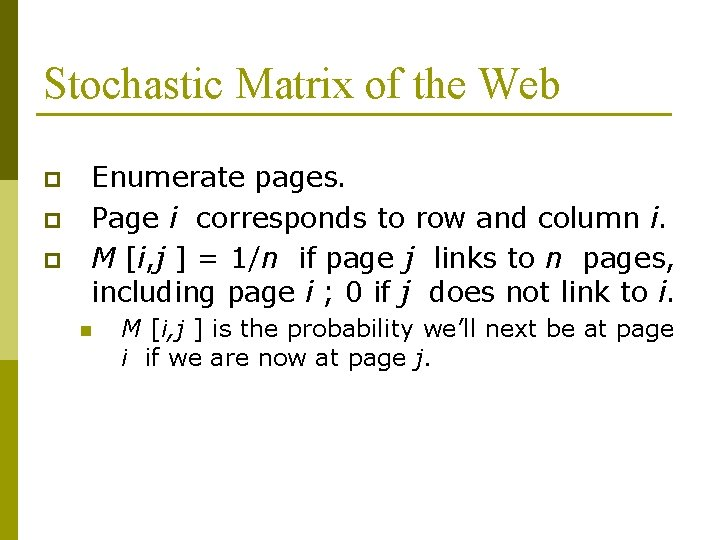Stochastic Matrix of the Web p p p Enumerate pages. Page i corresponds to row and column i. M [i, j ] = 1/n if page j links to n pages, including page i ; 0 if j does not link to i. n M [i, j ] is the probability we’ll next be at page i if we are now at page j.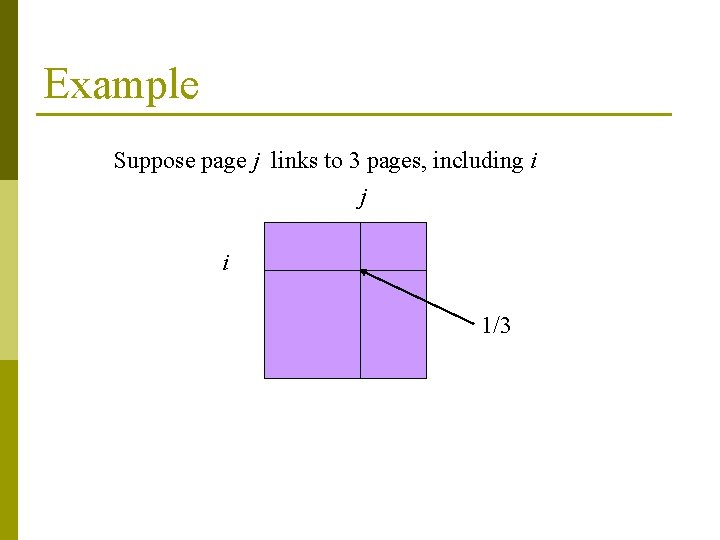Example Suppose page j links to 3 pages, including i j i 1/3Random Walks on the Web Suppose v is a vector whose i th component is the probability that we are at page i at a certain time. p If we follow a link from i at random, the probability distribution for the page we are then at is given by the vector M v. p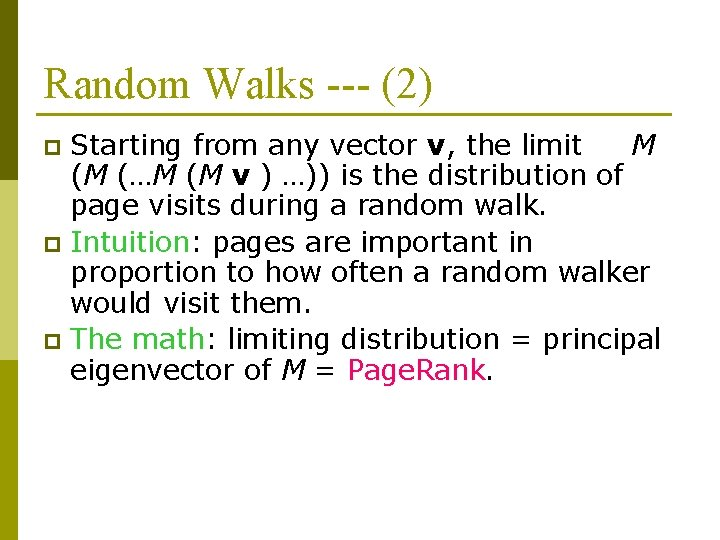Random Walks --- (2) Starting from any vector v, the limit M (M (…M (M v ) …)) is the distribution of page visits during a random walk. p Intuition: pages are important in proportion to how often a random walker would visit them. p The math: limiting distribution = principal eigenvector of M = Page. Rank. pExample: The Web in 1839 y a y 1/2 a 1/2 0 m 0 1/2 Yahoo Amazon M’soft m 0 1 0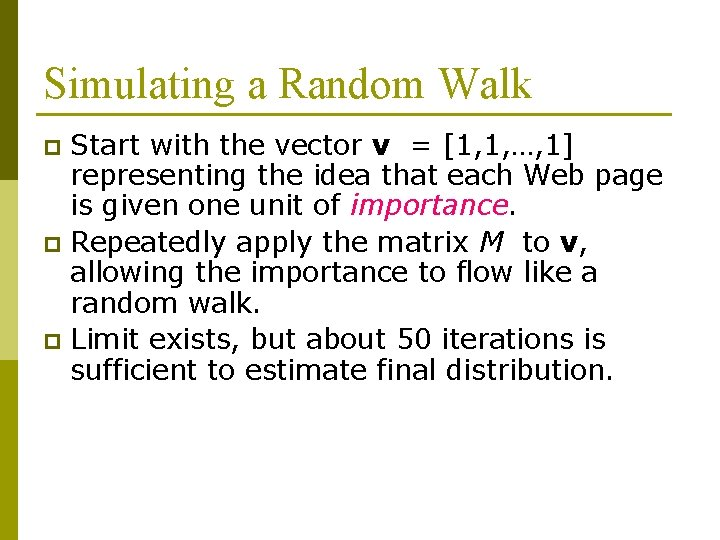Simulating a Random Walk Start with the vector v = [1, 1, …, 1] representing the idea that each Web page is given one unit of importance. p Repeatedly apply the matrix M to v, allowing the importance to flow like a random walk. p Limit exists, but about 50 iterations is sufficient to estimate final distribution. p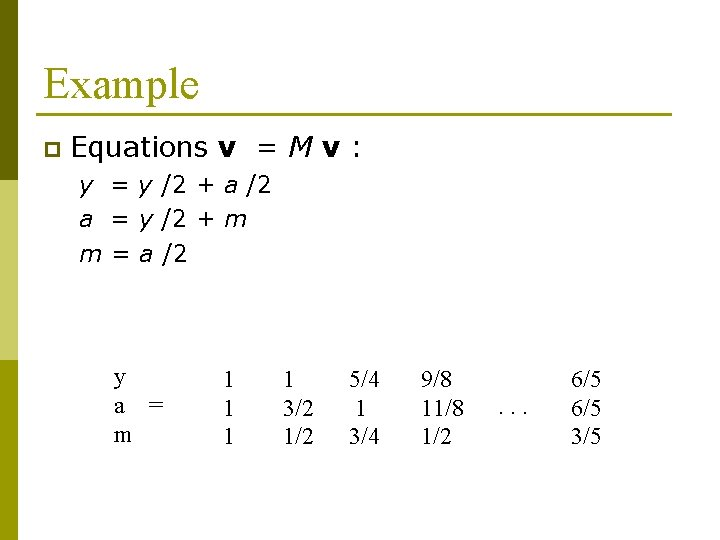Example p Equations v = M v : y = y /2 + a /2 a = y /2 + m m = a /2 y a = m 1 1 3/2 1/2 5/4 1 3/4 9/8 11/8 1/2 . . . 6/5 3/5Solving The Equations Because there are no constant terms, these 3 equations in 3 unknowns do not have a unique solution. p Add in the fact that y +a +m = 3 to solve. p In Web-sized examples, we cannot solve by Gaussian elimination; we need to use relaxation (= iterative solution). pReal-World Problems p Some pages are “dead ends” (have no links out). n p Such a page causes importance to leak out. Other (groups of) pages are spider traps (all out-links are within the group). n Eventually spider traps absorb all importance.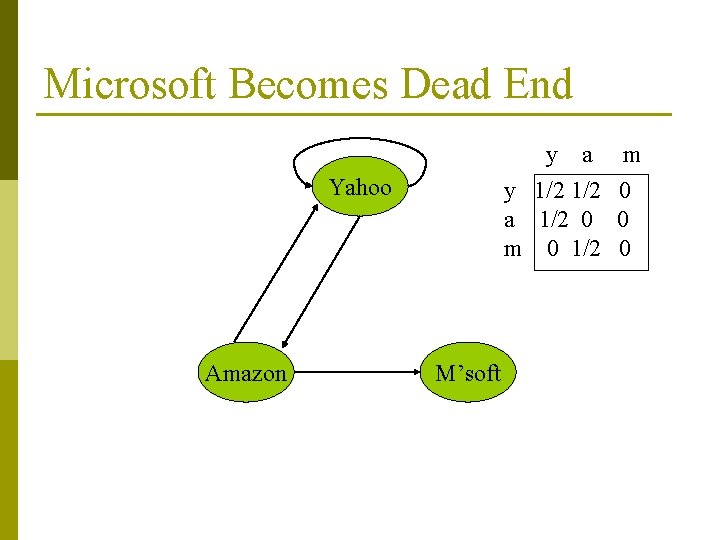Microsoft Becomes Dead End y a y 1/2 a 1/2 0 m 0 1/2 Yahoo Amazon M’soft m 0 0 0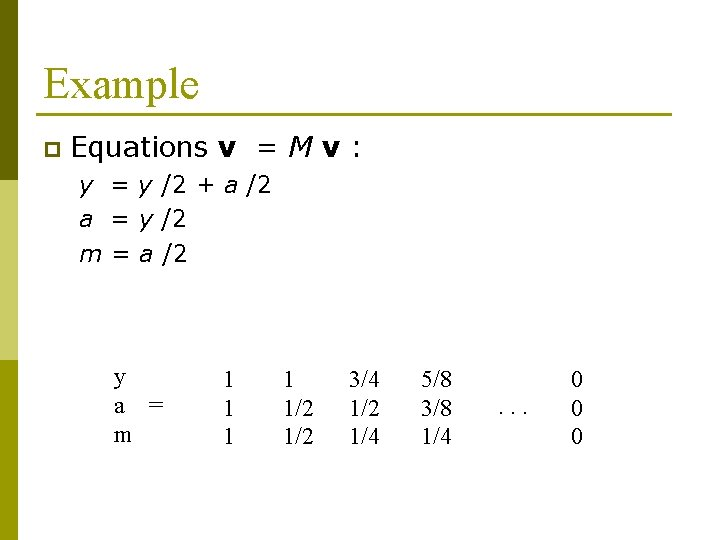Example p Equations v = M v : y = y /2 + a /2 a = y /2 m = a /2 y a = m 1 1 1/2 3/4 1/2 1/4 5/8 3/8 1/4 . . . 0 0 0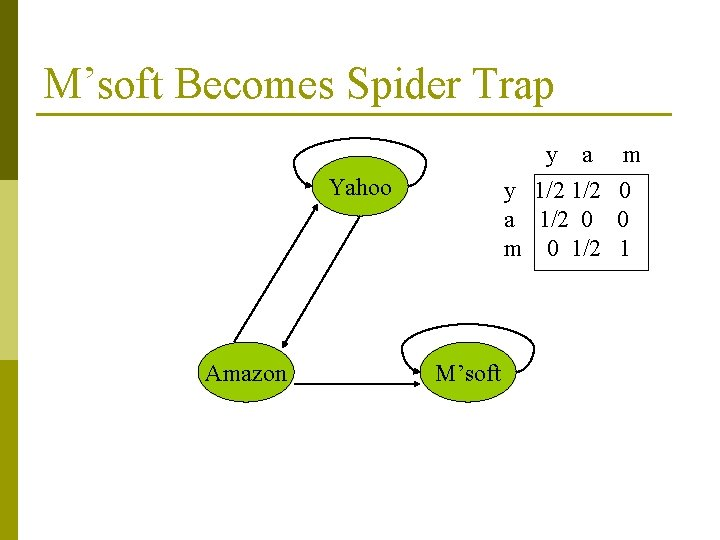M’soft Becomes Spider Trap y a y 1/2 a 1/2 0 m 0 1/2 Yahoo Amazon M’soft m 0 0 1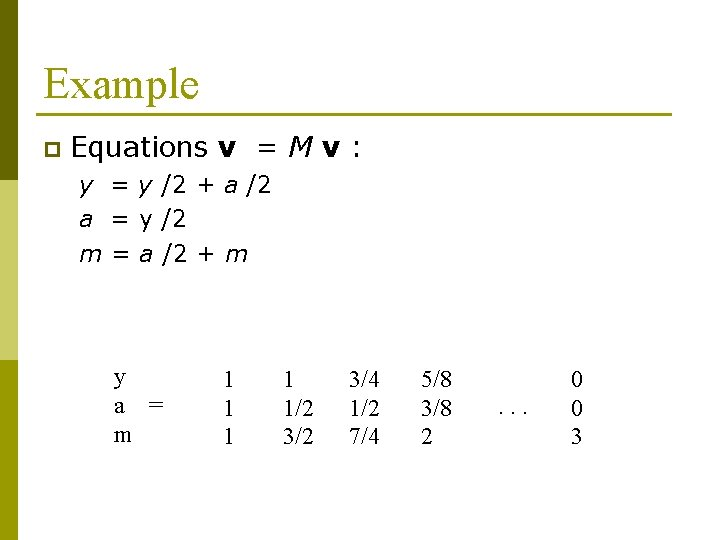Example p Equations v = M v : y = y /2 + a /2 a = y /2 m = a /2 + m y a = m 1 1 1/2 3/4 1/2 7/4 5/8 3/8 2 . . . 0 0 3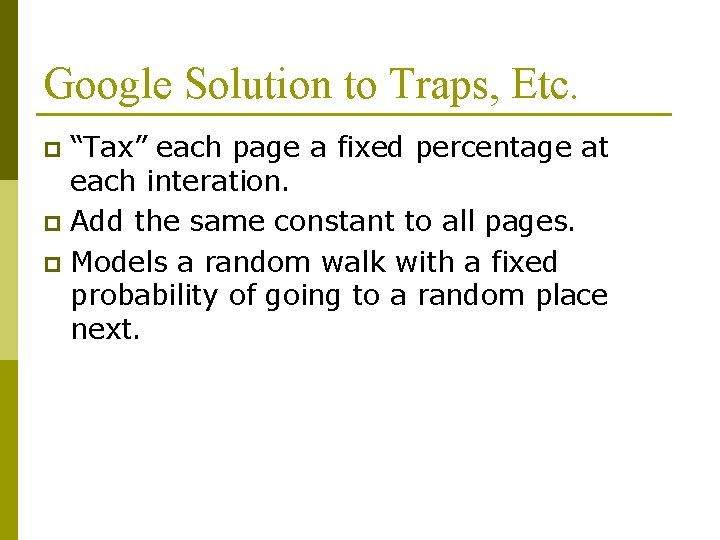Google Solution to Traps, Etc. “Tax” each page a fixed percentage at each interation. p Add the same constant to all pages. p Models a random walk with a fixed probability of going to a random place next. p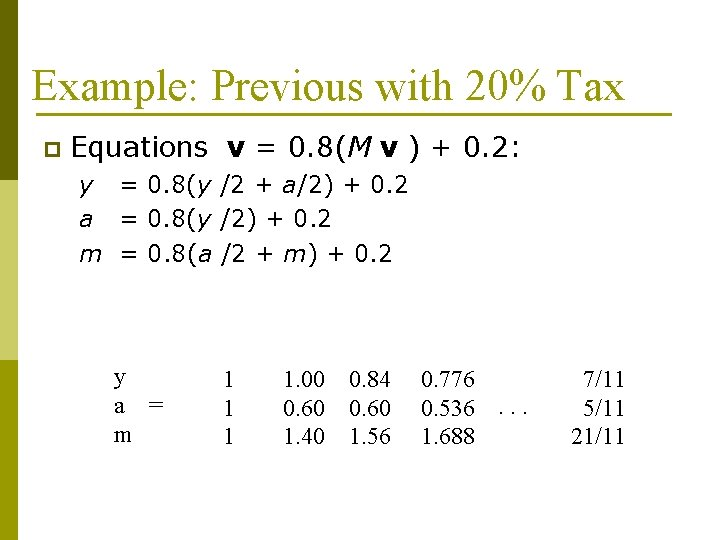Example: Previous with 20% Tax p Equations v = 0. 8(M v ) + 0. 2: y = 0. 8(y /2 + a/2) + 0. 2 a = 0. 8(y /2) + 0. 2 m = 0. 8(a /2 + m) + 0. 2 y a = m 1 1. 00 0. 60 1. 40 0. 84 0. 60 1. 56 0. 776 0. 536. . . 1. 688 7/11 5/11 21/11General Case In this example, because there are no dead-ends, the total importance remains at 3. p In examples with dead-ends, some importance leaks out, but total remains finite. pSolving the Equations Because there are constant terms, we can expect to solve small examples by Gaussian elimination. p Web-sized examples still need to be solved by relaxation. p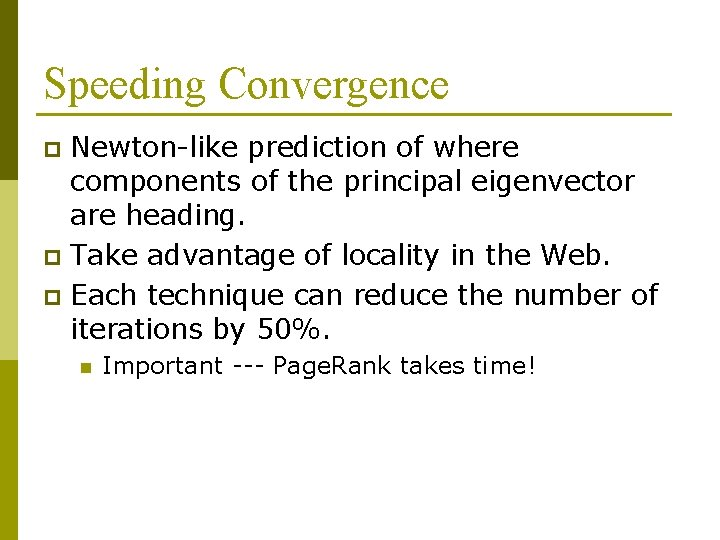Speeding Convergence Newton-like prediction of where components of the principal eigenvector are heading. p Take advantage of locality in the Web. p Each technique can reduce the number of iterations by 50%. p n Important --- Page. Rank takes time!